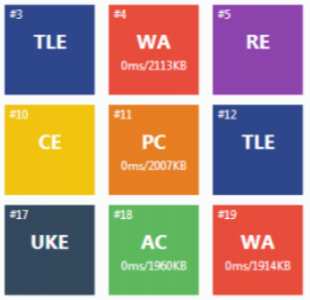# i2c物理层

• i2c通信总线可连接多个i2c通信设备，支持多个通信主机和多个通信从机。i2c通信只需要两条双向总线——SDA（串行数据线）和SCL（串行时钟线）。
SDA：用于传输数据
SCL：用于同步数据收发

• 每个连接到总线的设备都有一个独立地址，共7bit，主机正是利用该地址对设备进行访问

• i2c支持多主控，任何时间点都只能有一个主控。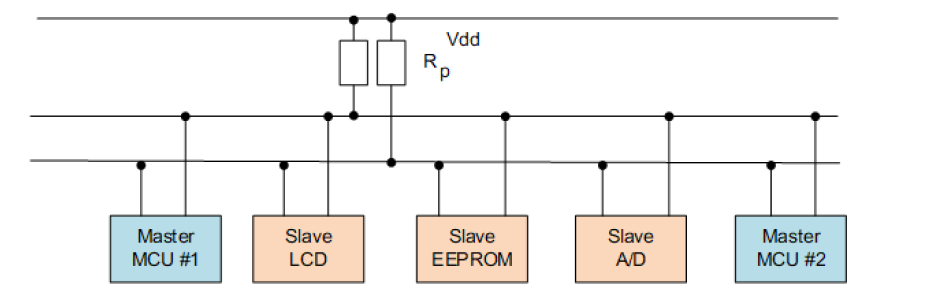• i2c器件的SDA引脚和SCL引脚是开漏电路形式，因此，SDA和SCL总线都需要连接上拉电阻，当总线空闲时，两条总线均为高电平。

• 各器件的SDA和SCL信号线在总线上都是线与关系。（即连接到总线上的任意器件输出低电平都会将总线信号拉低）

# 序言

1.读取数据
2.显示数据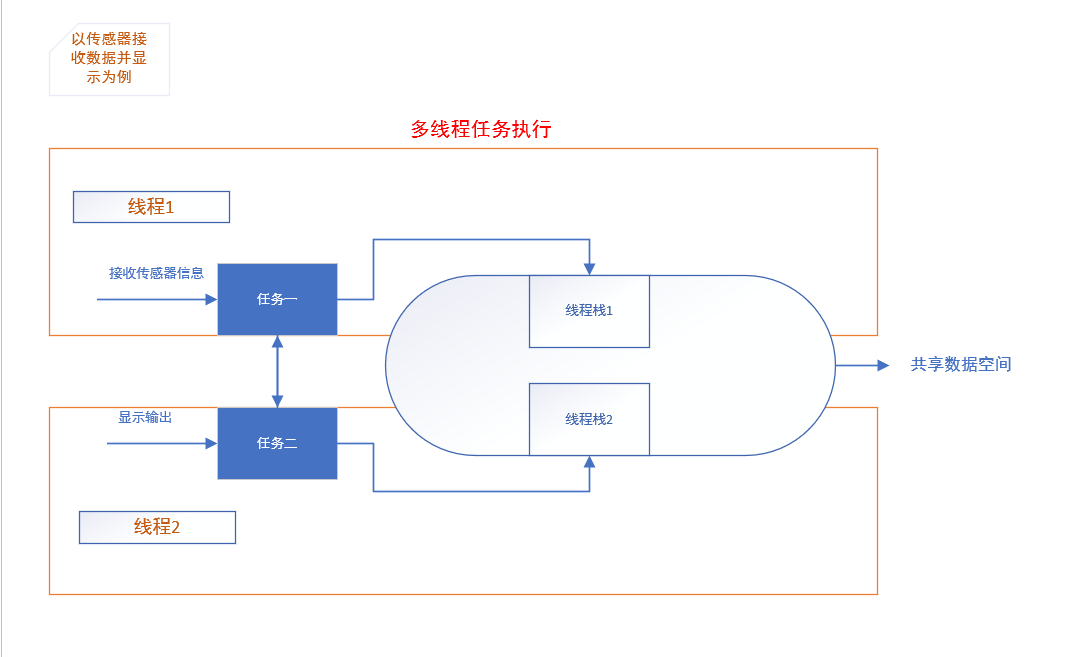上下文：当线程运行时，它会认为自己是以独占CPU 的方式在运行，线程执行时的运行环境称为上下文，具体来说就是各个变量和数据，包括所有的寄存器变量、堆栈、内存信息等。

# 问题描述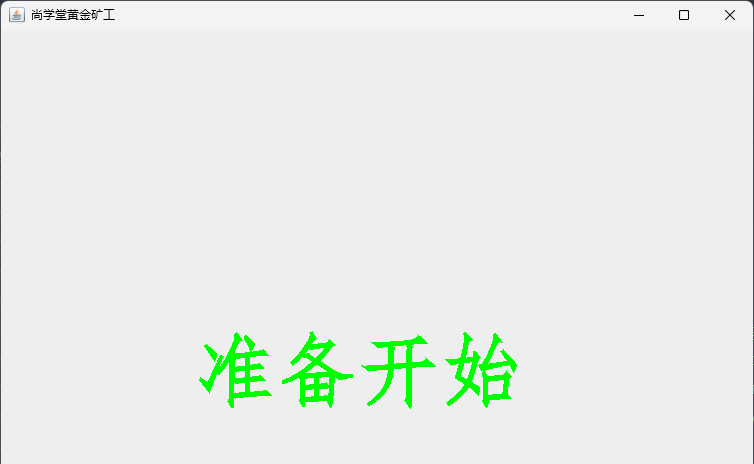# 表结构

• tb_student(sno,sn,dept,sex,birthday,polity)
• tb_score(sno,cno,score)
• tb_cource(cno,cn,ct,th)

# Problem 12 Highly divisible triangular number

The sequence of triangle numbers is generated by adding the natural numbers. So the 7th triangle number would be $1 + 2 + 3 + 4 + 5 + 6 + 7 = 28$. The first ten terms would be:

$$1, 3, 6, 10, 15, 21, 28, 36, 45, 55, …$$

Let us list the factors of the first seven triangle numbers: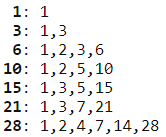We can see that $28$ is the first triangle number to have over five divisors.
What is the value of the first triangle number to have over five hundred divisors?

# Problem 13 Large sum

Work out the first ten digits of the sum of the following one-hundred $50$-digit numbers.

Data file see end

# 问题描述

FileNotFoundError: [Errno 2] No such file or directory: '/ProjectEuler/data/013.txt'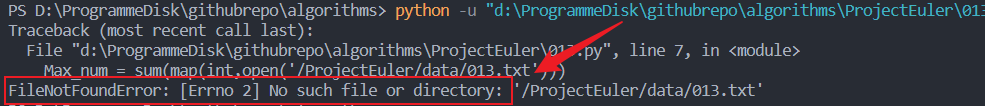👲👲作者主页：🔗杰森的博客
📒📒本文摘要用前端的知识实现立体滚动照片墙
💖💖感觉本文还不错的话，还请各位小伙伴👍点赞➕收藏⭐➕评论💭支持杰森呀✌️